# Remove Space Between Combined ggplot2 Plots in R (2 Examples)

In this R programming tutorial you’ll learn how to decrease the space between several combined ggplot2 graphics.

The page is structured as follows:

Let’s jump right to the examples!

## Example Data, Packages & Default Plot

Let’s first construct some example data in R:

```data <- data.frame(x = 1:6,           # Create example data frame
y1 = 1:6,
y2 = c(1, 4, 5, 3, 5, 2))
data                                  # Print example data frame```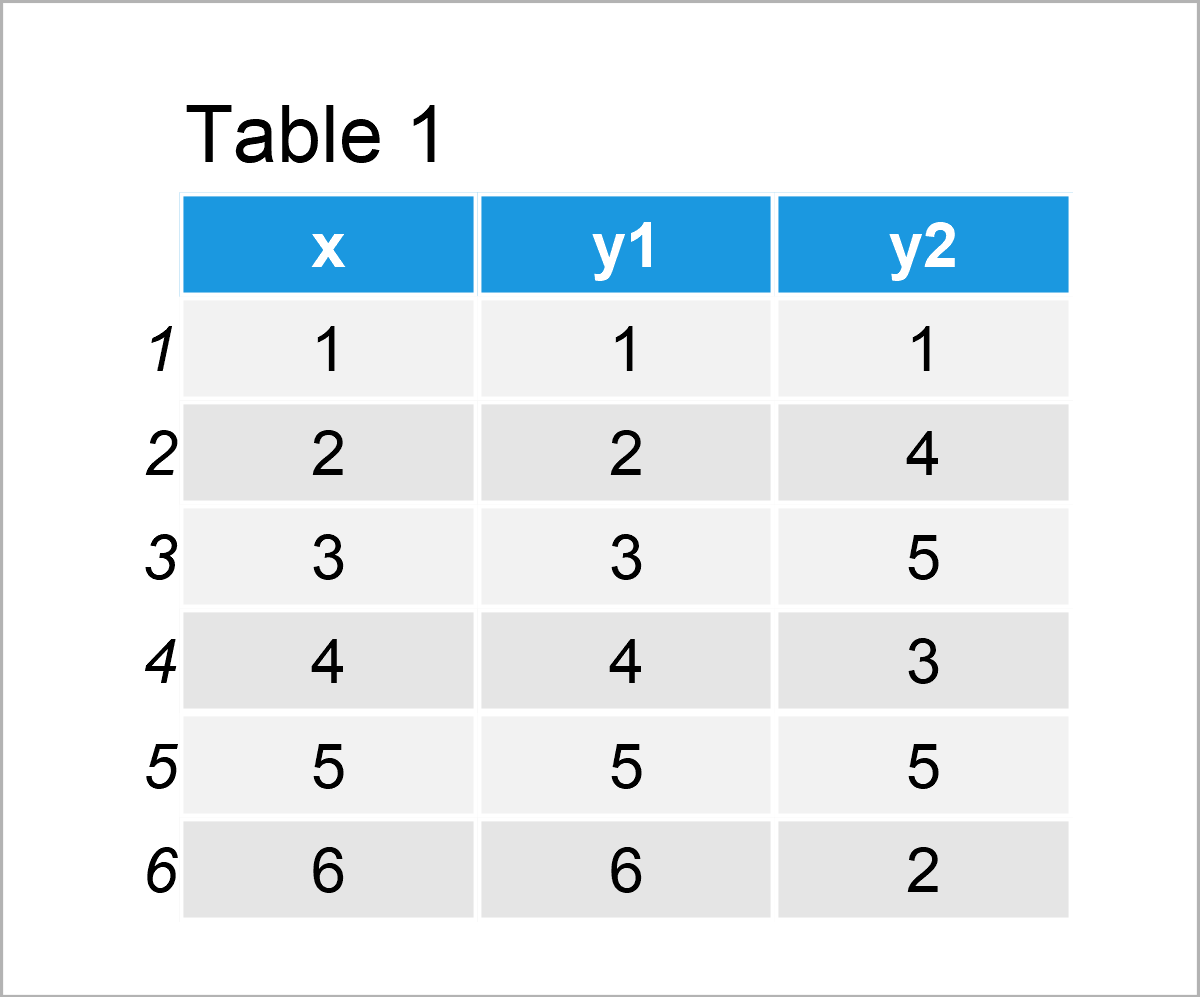As you can see based on Table 1, the example data is a data frame and contains six rows and three variables.

We also have to install and load the ggplot2 package, in order to draw ggplot2 plots:

```install.packages("ggplot2")           # Install & load ggplot2 package
library("ggplot2")```

As a next step, we can plot our data in two separate graphs as shown below::

```ggp1 <- ggplot(data, aes(x, y1)) +    # Create first ggplot2 plot
geom_point()
ggp1                                  # Draw first ggplot2 plot```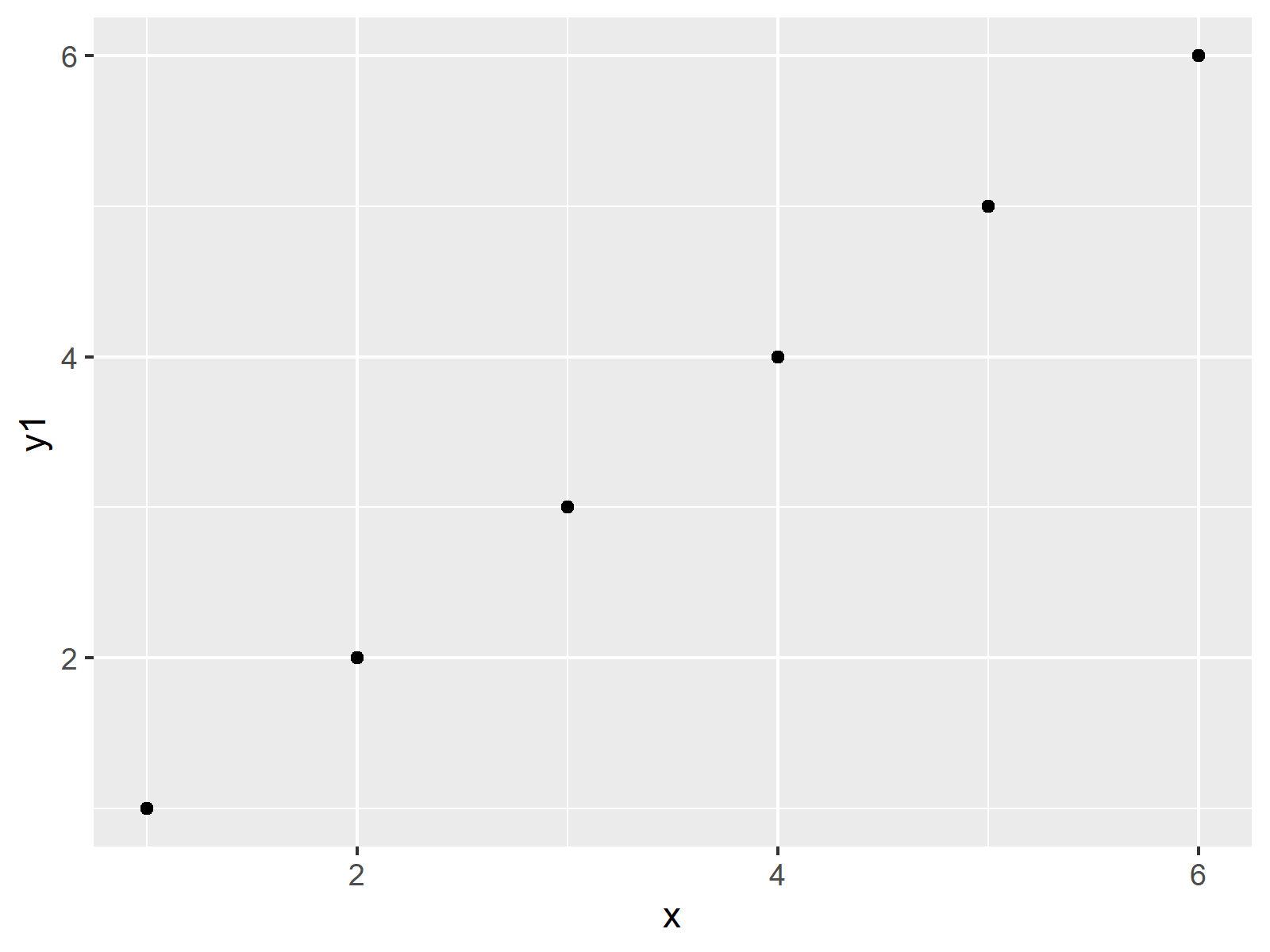```ggp2 <- ggplot(data, aes(x, y2)) +    # Create second ggplot2 plot
geom_line()
ggp2                                  # Draw second ggplot2 plot```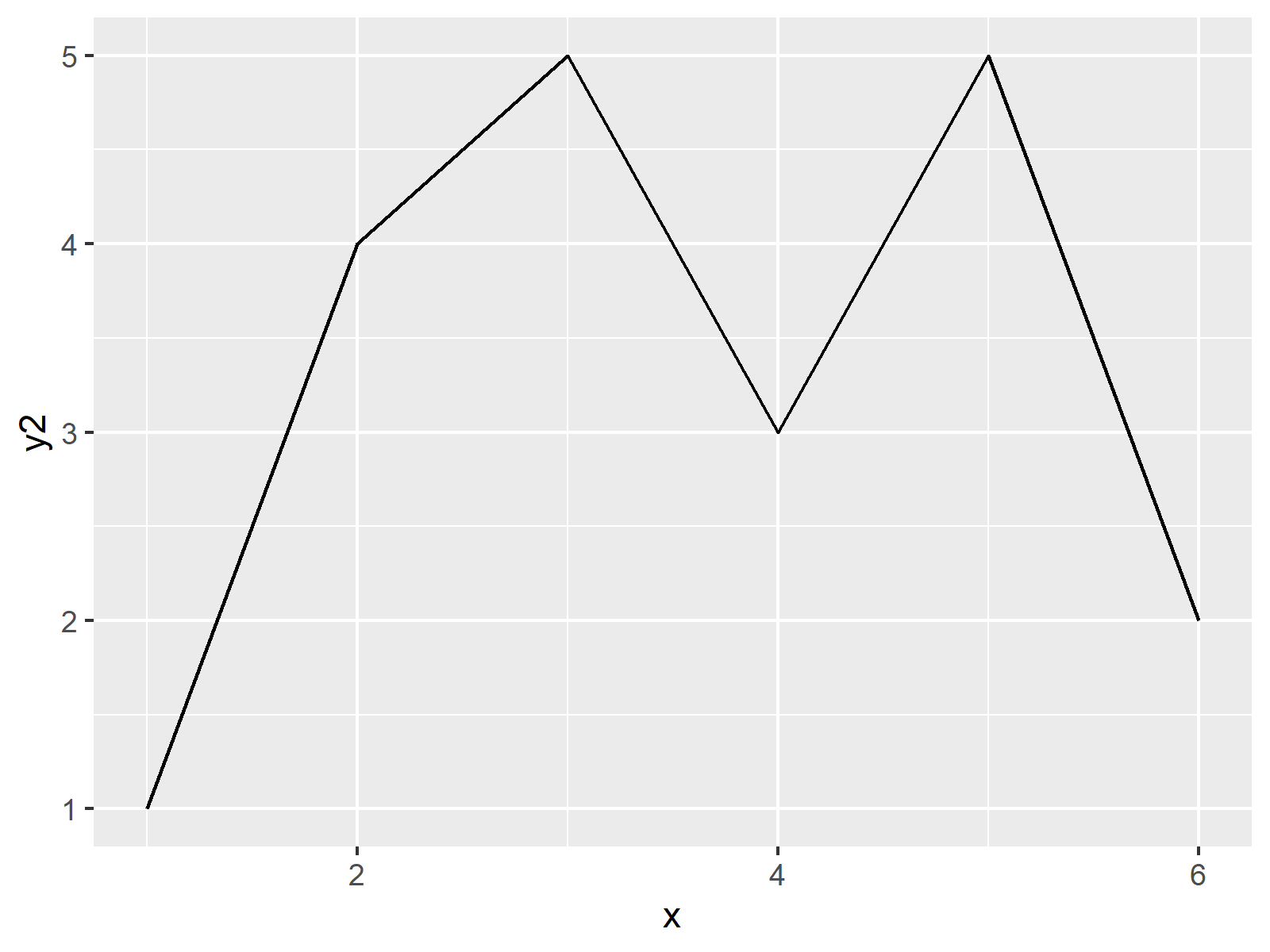The previous R code has created two plot objects called ggp1 and ggp2. Let’s arrange these plots in a grid layout!

## Example 1: Decrease White Space within Grid of ggplot2 Plots Created by gridExtra Package

Example 1 shows how to remove the space between multiple combined ggplot2 plots using the gridExtra package.

First, we need to install and load the gridExtra package:

```install.packages("gridExtra")         # Install gridExtra package

Next, we may apply the grid.arrange function to our two previously created plot objects to draw a plot composition:

`grid.arrange(ggp1, ggp2)              # Draw grid of default ggplot2 plots`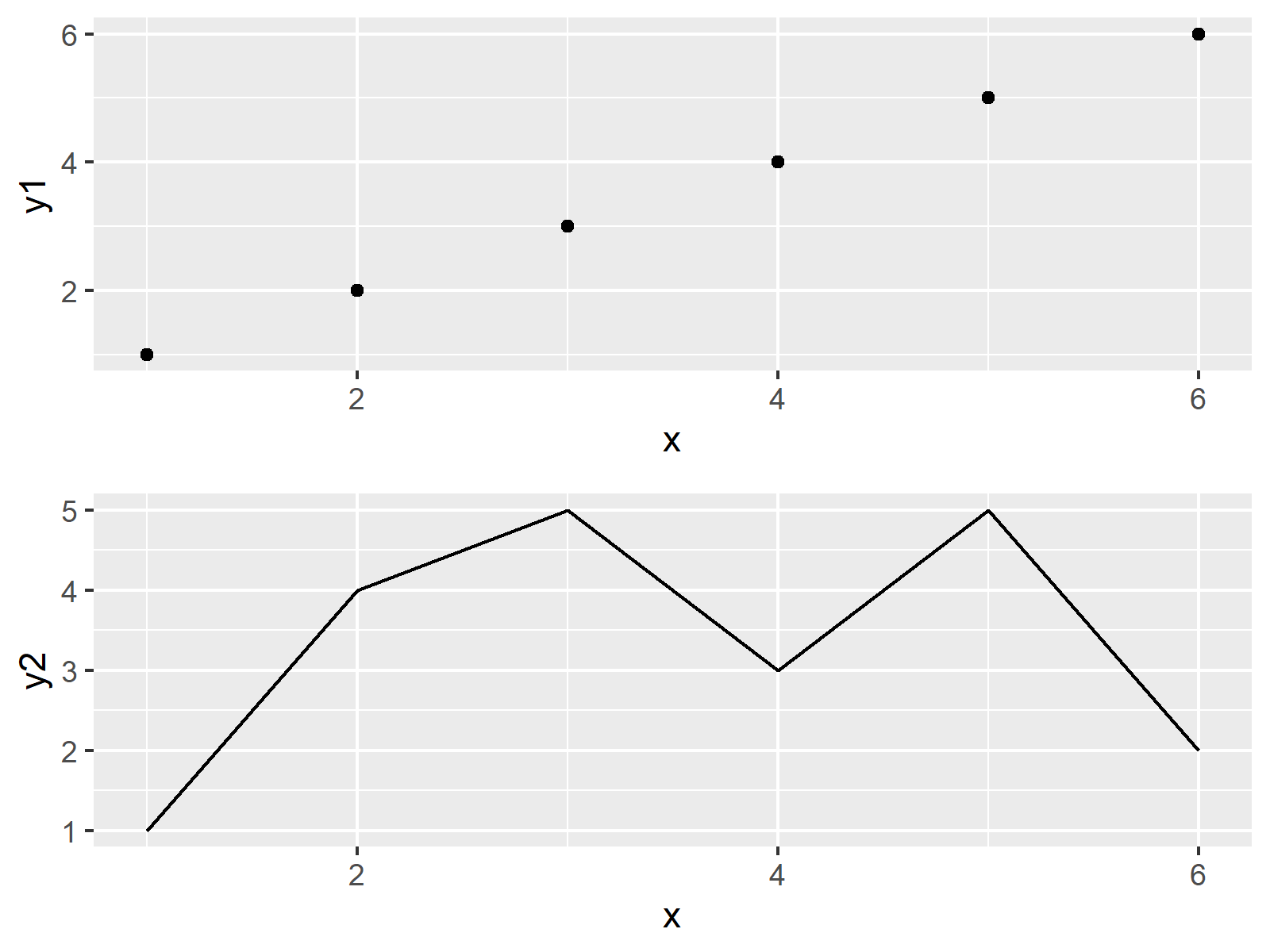Looks great, but the previous grid of plots contains a relatively large gap between two two graphics. Let’s decrease the space between our plots!

To achieve this, we first have to modify our two plot objects using the theme function.

The following R syntax removes the x-axis text, ticks, and title of the first plot. Furthermore, it changes the plot margins of this plot.

Note that 5.5pt is the default plot margin of a ggplot2 plot, i.e. in the code below we are only changing the area margin at the bottom of our graph.

```ggp1_mod <- ggp1 +                    # Modify margins of first ggplot2 plot
theme(axis.text.x = element_blank(),
axis.ticks.x = element_blank(),
axis.title.x = element_blank(),
plot.margin = unit(c(5.5, 5.5, 0, 5.5), "pt"))```

Next, we can use the theme function once again to adjust the margins at the top of our second plot:

```ggp2_mod <- ggp2 +                    # Modify margins of second ggplot2 plot
theme(plot.margin = unit(c(0, 5.5, 5.5, 5.5), "pt"))```

Finally, we can apply the grid.arrange function once again to draw our two plots side-by-side without the unnecessary space:

`grid.arrange(ggp1_mod, ggp2_mod)      # Draw grid of modified ggplot2 plots`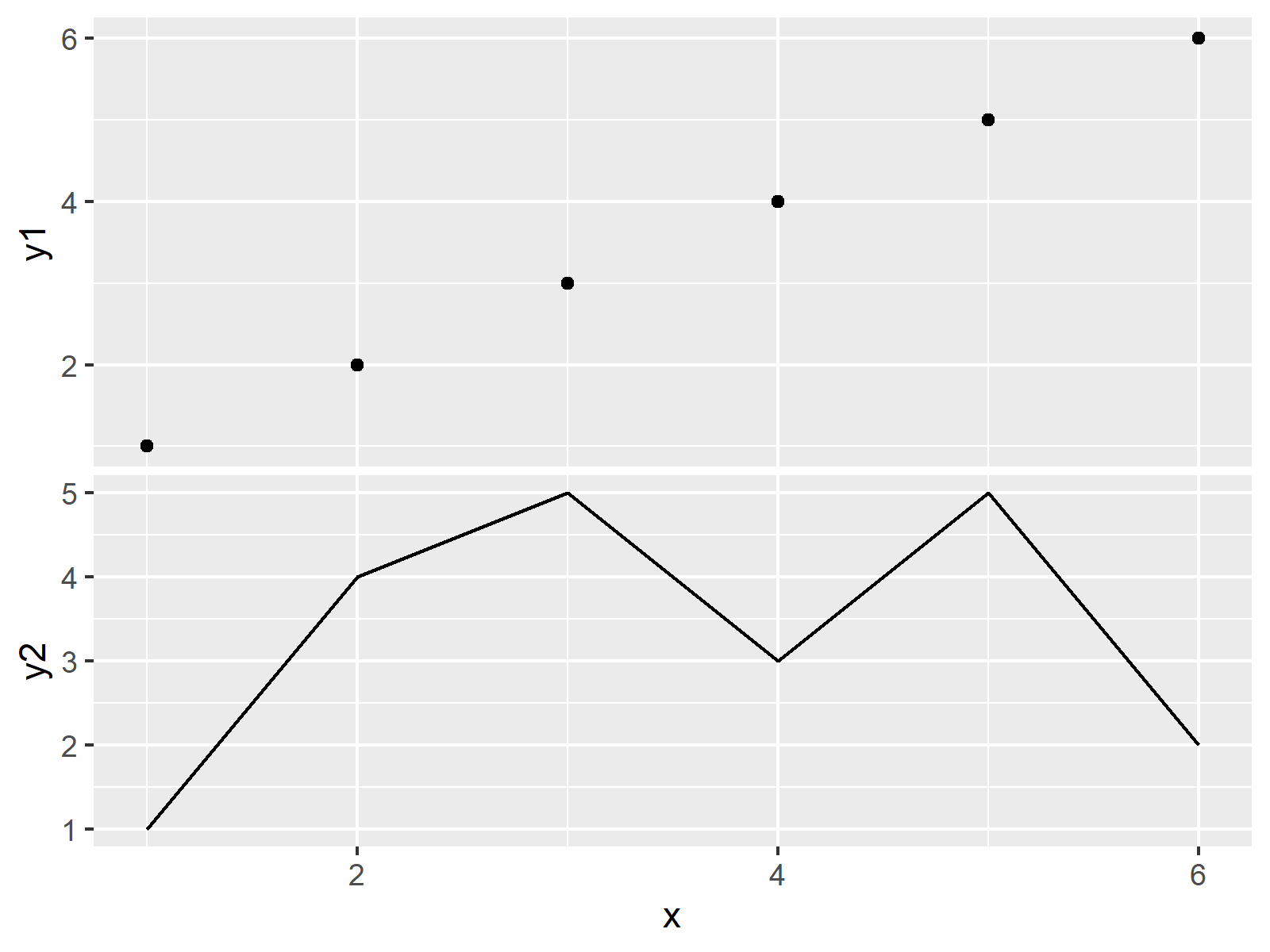## Example 2: Decrease White Space within Grid of ggplot2 Plots Created by patchwork Package

This section shows how to combine multiple ggplot2 plots with decreased space between the plots using the patchwork package.

We first need to install and load the patchwork package, in order to use the functions that are contained in the package:

```install.packages("patchwork")         # Install patchwork package

Next, we can use the two modified plot objects ggp1_mod and ggp2_mod that we have created in Example 1. However, this time we are drawing our grid layout using the patchwork package:

`ggp1_mod / ggp2_mod                   # Using patchwork package to draw plots`As you can see, the previous grid of plots looks very similar to the grid of plots created in Example 1. Whether you prefer to work with the gridExtra package or the patchwork package is a matter of taste.

## Video & Further Resources

Have a look at the following video on my YouTube channel. In the video, I’m explaining the content of this tutorial in RStudio:

In addition, you may want to read the related tutorials which I have published on my website. You can find a selection of tutorials on topics such as graphics in R, ggplot2, and labels below.